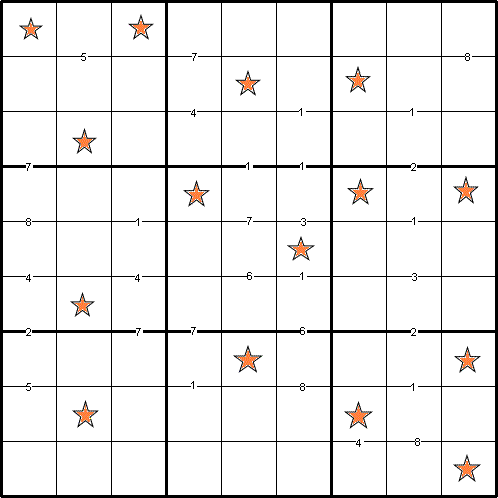#### You may also like### Eight Dominoes

Using the 8 dominoes make a square where each of the columns and rows adds up to 8### Magic Squares

An account of some magic squares and their properties and and how to construct them for yourself.### Magic Squares for Special Occasions

This article explains how to make your own magic square to mark a special occasion with the special date of your choice on the top line.

# Constellation Sudoku

##### Age 14 to 18 Challenge Level:
by Henry Kwok#### Rules of Constellation Sudoku

Like the standard sudoku, the object of the puzzle is to fill in the whole 9x9 grid with numbers 1 through 9 so that each row, each column, and each of the nine 3x3 squares must contain all the nine different numbers.

There are special clue-numbers placed on the border lines between selected pairs of adjacent squares of the grid. Each clue-number is the difference between the two numbers that should be in the adjacent squares just above and below that clue-number. For example, a clue-number 7 on the border line means that one of the possible answers in the square above the clue-number can be 9, 2, 8 or 1. If we choose 9 for the answer in the cell, it means that the answer in the square below it is 2. If we choose the answer 2, it means that the answer below it is 9.

The sudoku has two solutions when only clue-numbers are given, so a set of 15 digits {1, 2, 2, 3, 3, 4, 5, 5, 6, 7, 7, 8, 8, 8, 9} are also given to reduce those two solutions down to a single unique solution. The stars represents the positions for each digit from that set.

Hence, this puzzle is called 'Constellation Sudoku'.